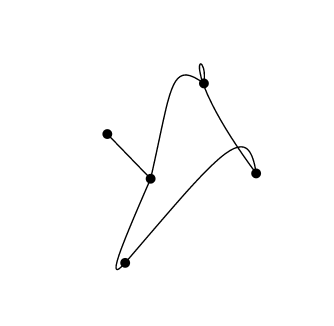# Graph Theory

I’m currently reading a book on graph theory.

A graph, in the mathematical sense, is a completely abstract object made up of sets. However, it definitely lends itself to visual representation, so I’m having a bit of fun making visualizations of some of the concepts.

## Math Lite

A graph (an abstract object – not to be confused with a representation of a graph!) is made up of two sets:

``````vertices = {v1, v2 ...}
edges = {e1,e2,..}
``````

However, the edge set may be empty. Each edge is incident to exactly two vertices.

Any two graphs are isomorphic if their vertex sets have a one-to-one relationship that preserves vertex adjacency. In other words, they may be represented differently but in the abstract they are the same.

## The Animation

I made the above animation to demonstrate that some very different looking graphs are in fact equal – you can see that no connections are ever forged or broken. Graph theory aside, it’s also interesting that some of the transitions I’ve animated appear to be taking place in 3 dimensions. I think this is especially true of transitions that cause edges to briefly take on dramatic angles, such as### SVG

The graph is created using `svg`, with one `path` element and five `circle` elements – one circle for each vertex. The segments of the path are all cubic bézier curves, à la `M0 100C55.2 100 100 55.2 100 0`, which I animated using javascript’s `requestAnimationFrame`.

### Javascript

I animate the graph by calling the following:

``````animate({start: shape1, end: shape2, total_frames:100});
``````

where `total_frames` is the number of frames I would like that animation to last, and `shape1/shape2` are arrays of coordinates, of the form `shape1 = [[67, 43], [64, -55],....]` etc.

Here’s the meat of that function:

``````function animate(object){
//`start` and `end` are both arrays of coordinates
var start = object.start;
var end = object.end;
var total_frames = object.total_frames;

var delta = determineDelta(start, end, total_frames);
startAnimationLoop(start, delta, total_frames);

}
``````

which calls

``````function startAnimationLoop(start, delta, max_count){
var currentPosition = start;
var count = 0;
function loop(){
draw(currentPosition);
setVertices(currentPosition);
currentPosition = getNextPosition(currentPosition, delta);
frame = requestAnimationFrame(loop);
if(count > max_count) cancelAnimationFrame(frame);
count++;
}
requestAnimationFrame(loop);
}
``````

and

``````function determineDelta(start, end, total_frames){
var deltas = [];
for(i=0; i < start.length; i++){
deltas[i] = [];
deltas[i] = -(start[i] - end[i])/total_frames;
deltas[i] = -(start[i] - end[i])/total_frames;
}
return deltas;
}
``````

which is simply determining which value to add to each point at each frame. The resultant value is the total change in position of each point (from start to end) divided by number of frames I want the animation to last. The function returns an array of values – one `delta[i] = [deltaX, deltaY]` for each point of the graph (including control points for cubic bézier curves).

The rest isn’t too interesting. The function `draw(array)` simply turns the coordinates array into proper svg path syntax and then sets the “d” attribute of a path element; `getNextPosition(array,delta)` increments the values of the coordinates by the amount determined for each point in `determineDelta(start, end, total_frames)`.

## That Circle

If you’re curious about how a perfect-seeming circle was represented using cubic bézier curves, check out this post.